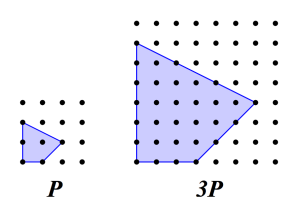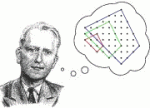# A Question of Counting

by Jonathan Kujawa

On November 8th everyone will be counting. Counting can be hard. Especially in messy real world situations like elections. But in pure mathematics we get to decide the questions in which we are interested. We can choose to count countable things. The secret to math is the art of asking “good” questions. A good question is one in which you can make progress and learn something you didn't know before.

Like Goldilocks, a mathematician looks for questions which are neither too easy nor too hard. An easy problem is boring, unenlightening, and not much fun — a 2x2x2 Rubik's cube of mathematics. But, like a 7x7x7 cube, a too difficult problem is also boring, unenlightening, and not much fun. The secret is to land somewhere in the middle.If we want to count, we should start by picking something easy to count, but with the promise of mystery. When I was in elementary school we spent hours and hours playing Dots and Boxes. This is the game in which you draw a grid of dots on a sheet of paper. The players take turns drawing horizontal and vertical line segments between dots trying to enclose boxes while blocking your friend from doing the same. The person with the most boxes at the end, wins. You can play it against a computer here.

If I had played less Dots and Boxes and paid more attention in my math classes, I would have learned that our initial dots were nothing but lattice points. These are the points in the xy-plane where both coordinates are an integer. So (1,3), (0,-6), and (2,127) are lattice points, but (1, 3/2) and (1.6, π) are not. Lattice points are easy to count in any geometric shape we may draw. For example, here is a 3×3 square with lattice points for corners:

Counting we see there are 12 lattice points on the sides of the square and 4 in the interior. Stuck in a boring math class for long enough, we might even notice that the area of the square, 9, is also what we get from 12/2 + 4 – 1. And that a 1×1 square has area 4/2 + 0 – 1 and that 2×2 square has area 8/2 + 1 – 1. In fact, it seems like for any square we draw if it has S lattice points on the sides and M lattice points on the interior, then the area always works out to exactly equal S/2 + M – 1. A strange coincidence, indeed! This is when our mathematical sense starts tingling. Coincidences are rare in math.

We furiously start drawing ever stranger shapes with corners at lattice points and straight lines connecting them, counting lattice points, and measuring areas. Even strange shapes like this one check out:It has 13 points on the sides and 3 interior points. Cutting up the shape into squares and triangles we work out that the area is exactly 13/2 + 3 – 1 = 8.5. We've just discovered Pick's Theorem. If you draw any polygon with lattice points for corners, the area will always exactly equal S/2 + M – 1! Remarkably, even though all we are doing is counting and calculating areas, it wasn't until 1899 that Pick discovered his result. This beautiful bit of mathematics could have been discovered by Euclid but, instead, hid in plain sight for more than two thousand years. Wolfram Demonstrations Project has a great free app if you'd like to see Pick's Theorem in action.

As mathematically inclined folks we are never satisfied. Pick's theorem is for polygons. Surely other geometric shapes are worth trying. Gauss himself asked if there is a nice formula for the number of lattice points inside a circle of radius r. As we saw last year here at 3QD, Gauss was a master of counting. It is no wonder he took a stab at counting lattice points. What shape could be more simple than the good ol' circle? For example, the circles of radius 1, 2, and 3 contain a total of 5, 13, and 29 lattice points:Despite people's best efforts there doesn't seem to be a Pick's Theorem for circles. In fact, it's worse than that. We don't even know how to precisely relate the number of lattice points in a circle to its area. Since each square unit of area has, more or less, one lattice point, we should expect that the number of lattice points in a circle of radius r to be close to the area of the circle. That is, if N(r) is the number of lattice points in a circle of radius r, then we should expect,

N(r)= πr2 + E(r),

where E(r) is the error we have to add or subtract from the area to get the number of lattice points. Remarkably, we still don't have a good description of that error term! Gauss himself proved that |E(r)| is less than 2√2πr (that is, not more than ~8.89r). Nowadays we know that |E(r)| is bounded by Crt where C is some positive constant and t is between 1/2 and 131/208 (that is, between 0.5 and ~0.63). Which might sound pretty good until you calculate and discover that when r is, say, a googolplex, the gap caused by this uncertainty is truly brobdinagian! It is an open problem to improve the bounds on t. If you want to play with it yourself, Wolfram has an app for that.

Even though Gauss came before Pick, clearly he asked the much harder question. If we want to take Pick's theorem in a different direction, how about we go up a dimension? You can talk about lattice points in three dimensional space — these are the points who have x, y, and z coordinates given by integers. We can play Pick's game and consider the 3D shapes obtained by connecting lattice points in 3 dimensional space. These shapes are called polytopes. Is there a nice Pick-like formula which can calculate the volume of a polytope from a count of its lattice points?Reeve's tetrahedra. Click to animate.

After playing with polytopes, you might stumble upon Reeve's tetrahedra. These are the polygons you make if you connect the points (0,0,0), (1,0,0), (0,1,0), and (1,1,r), where r can be 1,2,3,4,… Reeve discovered these in 1957 while trying to work out a 3D version of Pick's theorem. From the point of view of Pick's theorem, these shapes are a disaster. The only lattice points they contain are the 4 at the corners, but as r gets larger and larger, the volume grows and grows! This puts the nail in the coffin on any hope of having a formula for volume of polytopes in terms of their lattice points. Lest you hold out hope that 3D is the only problematic case, I should warn you similar beasts also live in dimensions four and higher.

But just because something is impossible doesn't mean we should give up. In the 1960s Ehrhart had the very clever idea to turn Pick's theorem inside out. Instead of trying to obtain a formula for volume in terms of the number lattice points, why not try to obtain a formula for the number of lattice points in terms of the volume?

Ehrhart's second clever idea was to trade the problem of counting the lattice points in single polytope for the seemingly harder problem of counting the lattice points in entire families of polytopes all in one go. It may seem like this will only add to our difficulties, but through long experience mathematicians have found that oftentimes it is actually easier to find formulas for counting in groups. We saw a bit of this here at 3QD when we crossed paths with generating functions. Seemingly random strings of numbers can coalesce into beautiful formulas when tied together in just the right way.

In Earhart's case, he asked the following question: if you start with a polytope P and double, triple, quadruple,… it in size, is there a nice formula for the number of lattice points in this chain of ever larger polytopes? In general, starting with a polytope P and writing 2P, 3P, …, nP, … for its double, triple, etc., can we find a formula which calculates, L(nP), the number of lattice points in nP?Image from .

Amazingly enough, the answer is yes! If P is a polytope in d dimensional space, then there is a single polynomial of degree d with variable n, f(n), which will tell you how many lattice points are in nP! Exactly what it looks like depends on which polytope you start with, of course. For example, if P is the 1×1 square, then the number of lattice points in nP is given by f(n)=(n+1)2. More generally, if P is the d-dimensional hypercube with side lengths 1, then the number of lattice points is given by f(n)=(n+1)d.

In general Earhart polynomials can get quite complicated. Nevertheless we can say a few things. For example, the leading coefficient is always the volume of P and the constant coefficient is always 1. Some of the other coefficients are also known to have geometric interpretations, but others are still mysterious.Ehrhart pondering polytopes. Click to animate .

Never satisfied, mathematicians have asked if you can push Ehrhart's theory even further. If you start with a polytope in which corners are allowed to have fractional numbers as coordinates and not just integers, then you can still count the number of lattice points and now the formulas are given by several different polynomials where the polynomial you use depends on which n you are interested in.

That's about the limit of what we know. If you make a polytope with corners which have irrational coordinates (like e, √2, or π), then all bets are off. There doesn't seem to be any nice formulas for counting lattice points in these polytopes. At least none that we know of. But then again, maybe we're just not asking the right question.

 Image from here. These are slides from a nice talk by Stanley on Ehrhart theory if you'd like to learn more.

 Image from here.